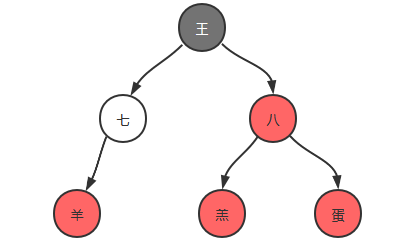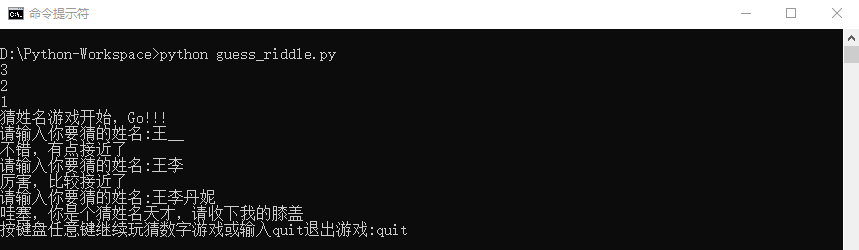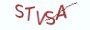Python基础教程

# 程序难点分析```name = "王八蛋"
trie = {}
trie[name] =  {}
trie[name][name] =  {}
trie[name][name][name] =  {}```

# 程序完整源码

```# __author__ = 薯条老师
# 微信公众号 =  薯条编程
import time

def countdown(seconds=3, message=""):
"""
:param seconds: 倒数的秒数
:param message:  倒计时结束后输出的提示信息
:return:
"""
for _ in range(seconds, 0, -1):
_, _ = print(_), time.sleep(1)
else:
print(message)

def build_lookup_table(data):
"""
:param data: a set of names, e.g: {"王祖贤", "李嘉欣"}
:return: lookup table e.g:{'王': {'祖': {'贤': {}}}, '李': {'嘉': {'欣': {}}}}
"""
lookup_table = {}
for name in data:
lookup_table_ = lookup_table
for char in name:
if char not in lookup_table_:
lookup_table_[char] = {}
lookup_table_ = lookup_table_[char]
return lookup_table

def guess_name():
stars = {"王祖贤", "李嘉欣", "李嘉诚", "刘德华", "叶倩文", "叶倩倩", "王李丹妮"}
# 构造一个查找表
lookup_table = build_lookup_table(stars)
countdown(3, "猜姓名游戏开始，Go!!!")
messages = {0: "不要瞎猜好吗?",  1: "不错，有点接近了",  2: "厉害，比较接近了",
66: "哇塞，你是个猜姓名天才，请收下我的膝盖"}
end = 2
while True:
name = input("请输入你要猜的姓名:____\b\b\b\b")
if name in stars:
print(messages)
if input("按键盘任意键继续玩猜数字游戏或输入quit退出游戏:____\b\b\b\b").lower() == "quit":
break
else:
lookup_table_ = lookup_table
index = 0
for word in name:
if word in lookup_table_:
lookup_table_ = lookup_table_[word]
index = index+1 if index < end else index
else:
break
print(messages[index])
if __name__ == "__main__":
guess_name()```# 最具实力的小班培训

`(1) Python后端工程师高薪就业班，月薪11K-18K，免费领取课程大纲(2) Python爬虫工程师高薪就业班，年薪十五万，免费领取课程大纲(3) Java后端开发工程师高薪就业班，月薪11K-20K, 免费领取课程大纲(4) Python大数据分析，量化投资就业班，月薪12K-25K,免费领取课程大纲`## 注册账号